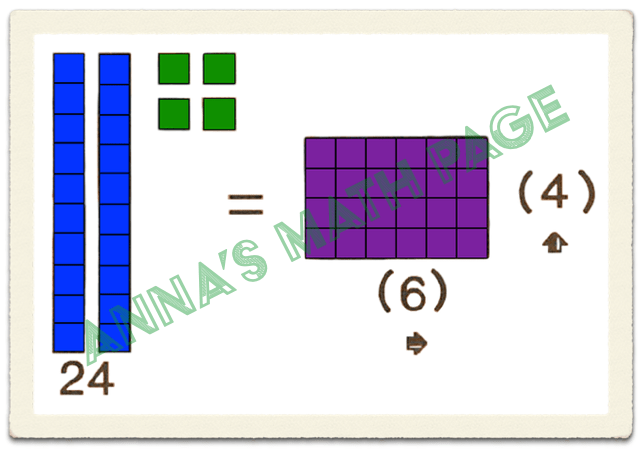# ArithmeticMathematics is the study of numbers. We count numbers.

In arithmetic we will practice addition multiplication, division, subtraction, fractions, decimals, and percents.